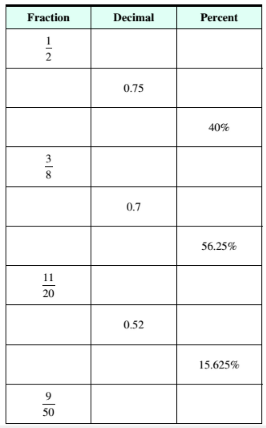Chapter 9.3, Problem 6ESMathematical Excursions (MindTap C...

4th Edition
Richard N. Aufmann + 3 others
ISBN: 9781305965584

Solutions

Chapter
SectionMathematical Excursions (MindTap C...

4th Edition
Richard N. Aufmann + 3 others
ISBN: 9781305965584
Textbook Problem

Complete the table of equivalent fractions, decimals, and percent’s.To determine

To calculate: The decimal and percent for the fraction 38 .

Explanation

Given Information:

Fraction, 38

Formula used:

To write fraction as decimal, perform division of the numerator by the denominator.

Calculation:

Consider the fraction,

38

Perform division of numerator by the denominator to write fraction 38 as decimal,

38=0

Still sussing out bartleby?

Check out a sample textbook solution.

See a sample solution

The Solution to Your Study Problems

Bartleby provides explanations to thousands of textbook problems written by our experts, many with advanced degrees!

Get Started

Find more solutions based on key concepts